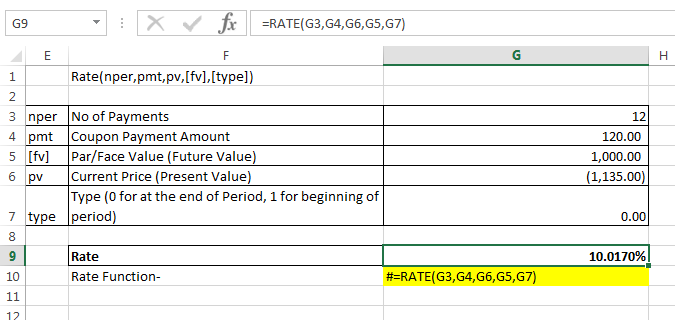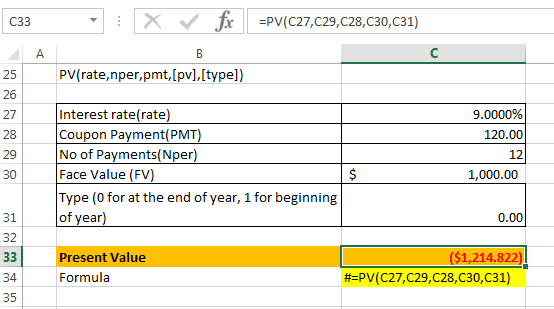In: Finance

# The 12​-year, ​$1,000 par value bonds of Waco Industries pay 12percent interest annually. The market... The 12-year,$1,000 par value bonds of Waco Industries pay 12 percent interest annually. The market price of the bond is $1,135, and the market's required yield to maturity on a comparable-risk bond is 9 percent. Compute the bond's yield to maturity. 1. Determine the value of the bond to you given the market's required yield to maturity on a comparable-risk bond. ## Solutions ##### Expert Solution Face Value of Bond =$1000

Annual Coupon Payment = $1000*12% =$120

No of years to maturity = 12 years

a). Current Market Price of Bond = $1135 Calculating the Yield to Maturity of Bond using Excel "RATE" Function:-So,the Yield to Maturity(YTM) of Bond is 10.02% b). Market Yield to Maturity on comparable bond is 9% Calculating the Price of Bond using excel "PV" function:-So, Price of Bond based on Market Yield to Maturity on comparable bond is$1,214.82

## Related Solutions

##### The 16​-year, ​$1,000 par value bonds of Waco Industries pay 11 percent interest annually. The market... The 16​-year, ​$1,000 par value bonds of Waco Industries pay 11 percent interest annually. The market price of the bond is ​$945​, and the​ market's required yield to maturity on a​ comparable-risk bond is 10 percent. a. Compute the​ bond's yield to maturity. b. Determine the value of the bond to you given the​ market's required yield to maturity on a​ comparable-risk bond. c. Should you purchase the​ bond? ##### The 11​-year, ​$1,000 par value bonds of Waco Industries pay 9 percent interest annually. The market...
The 11​-year, ​$1,000 par value bonds of Waco Industries pay 9 percent interest annually. The market price of the bond is ​$1,095​, and the​ market's required yield to maturity on a​ comparable-risk bond is 6 percent. a.  Compute the​ bond's yield to maturity. b.  Determine the value of the bond to you given the​ market's required yield to maturity on a​ comparable-risk bond. c.  Should you purchase the​ bond?
##### The 19​-year, ​$1000 par value bonds of Waco Industries pay 8 percent interest annually. The market... The 19​-year, ​$1000 par value bonds of Waco Industries pay 8 percent interest annually. The market price of the bond is ​$1105​, and the​ market's required yield to maturity on a​ comparable-risk bond is 5 percent. a.Compute the​ bond's yield to maturity. b.Determine the value of the bond to you given the​ market's required yield to maturity on a​ comparable-risk bond. c.Should you purchase the​ bond? a.What is your yield to maturity on the Waco bonds given the current market... ##### The 12-year,$1,000 par value bonds of Smith Industries pay 7 percent interest annually. The market...
The 12-year, $1,000 par value bonds of Smith Industries pay 7 percent interest annually. The market price of the bond is$885, and the market’s required yield to maturity on a​ comparable-risk bond is 10 percent. What is your yield to maturity on the Smith bonds given the current market price of the​ bonds? Round to two decimal places. What should be the value of the Smith bonds given the​ market's required yield to maturity on a​comparable-risk bond? Round to...
##### (Bond valuation​ relationships)The 14​-year, $1,000 par value bonds of Waco Industries pay 11 percent interest annually.... (Bond valuation​ relationships)The 14​-year,$1,000 par value bonds of Waco Industries pay 11 percent interest annually. The market price of the bond is $1,115​, and the​ market's required yield to maturity on a​ comparable-risk bond is 8 percent. a. Compute the​ bond's yield to maturity. b. Determine the value of the bond to you given the​ market's required yield to maturity on a​ comparable-risk bond. c. Should you purchase the​ bond? 1) What is your yield to maturity on the... ##### The 11-year,$1,000 par value bonds of Witt Industries pay 7 percent interest annually. The market...
The 11-year, $1,000 par value bonds of Witt Industries pay 7 percent interest annually. The market price of the bond is$865, and the market’s required yield to maturity on a comparable-risk bond is 10 percent. A. What is your yield to maturity on the Witt bonds given the current market price of the bonds? _% Please round to two decimal places. B. What should be the value of the Witt bonds given the market's required yield to maturity on...
##### ​Fingen's 12​-year, ​$1,000 par value bonds pay 15 percent interest annually. The market price of the... ​Fingen's 12​-year, ​$1,000 par value bonds pay 15 percent interest annually. The market price of the bonds is ​$880 and the​ market's required yield to maturity on a​ comparable-risk bond is 16 percent. a. Compute the​ bond's yield to maturity. b. Determine the value of the bond to​ you, given your required rate of return. c. Should you purchase the​ bond? ##### Fingen's 15​-year, ​$1,000 par value bonds pay 12 percent interest annually. The market price of the...
Fingen's 15​-year, ​$1,000 par value bonds pay 12 percent interest annually. The market price of the bonds is ​$1,100 and the​ market's required yield to maturity on a​ comparable-risk bond is 9 percent. a.  Compute the​ bond's yield to maturity. b.  Determine the value of the bond to​ you, given your required rate of return. c.  Should you purchase the​ bond?
##### ExxonMobil ​12 year bonds pay 9 percent interest annually on a ​$1,000 par value. If the... ExxonMobil ​12 year bonds pay 9 percent interest annually on a ​$1,000 par value. If the bonds sell at $775 what is the​ bonds' expected rate of​ return? The​ bonds' expected rate of return is ? ##### Fingen's 15​-year, ​$1,000  par value bonds pay 11 percent interest annually. The market price of the bonds...
Fingen's 15​-year, ​$1,000 par value bonds pay 11 percent interest annually. The market price of the bonds is ​$920 and the​ market's required yield to maturity on a​ comparable-risk bond is 14 percent. a.  Compute the​ bond's yield to maturity. b.  Determine the value of the bond to​ you, given your required rate of return. c.  Should you purchase the​ bond?